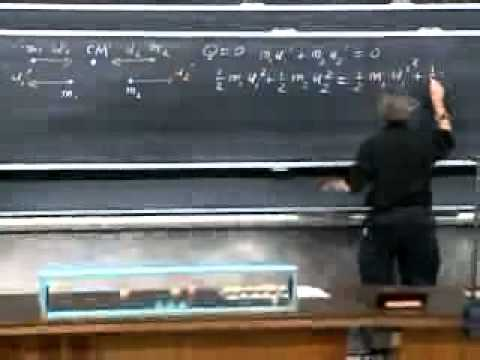# Perfectly Elastic Physics Help?

A ball of mass 0.340 kg moving east (+x direction) with a speed of 3.10 m/s collides head-on with a 0.540 kg ball at rest. If the collision is perfectly elastic, what will be the speed and direction of each ball after the collision?

Tried doing it myself, did NOT work...HELP

Relevance

There is a nifty little trick, that you can do, by switching reference frames. See the following video for an explanation:&feature=player_detailpage#t=1456s

The given speeds, are in the Lab reference frame. Use the letter v to talk about velocity in the lab reference frame.

Data:

m1 = 0.34 kg

m2 = 0.54 kg

v1i = 3.1 m/s

v2i = 0 m/s

v1i and v2i, are the initial velocities in the lab reference frame.

The special reference frame is the center of mass reference frame. Use u to talk about velocity

The velocity of the center of mass, in the lab reference frame is:

vcm = (v1i*m1 + v2i*m2)/(m1 + m2)

vcm = 1.198 m/s

To translate to the center of mass reference frame:

u1i = v1i - vcm

u2i = v2i - vcm

u1i = 1.902 m/s

u2i = -1.198 m/s

The velocity of the center of mass in the center of mass reference frame, will remain constant, and will remain zero. It will remain, because momentum is conserved in the absence of net external forces.

The way that both momentum and kinetic energy get conserved, is that the velocities will REVERSE direction in the center of mass reference frame. This is a nice shortcut, because we can avoid solving a big quadratic equation.

This is for the SPECIAL CASE of a completely elastic collision.

For a completely inelastic collision (not our concern today), they will collide and come to rest in the center of mass reference frame.

u1f = -u1i

u2f = -u2i

u1f = -1.902 m/s

u2f = 1.198 m/s

And translate back to the lab reference frame:

v1f = u1f + vcm

v2f = u2f + vcm

Results:

v1f = -0.7045 m/s

v2f = 2.395 m/s

The negative sign on v1f, indicates that ball 1 will reverse direction in the laboratory reference frame, due to this collision. It will be moving west, after the collision.

Confirm for yourself that both momentum and kinetic energy are conserved.